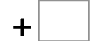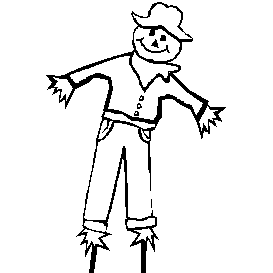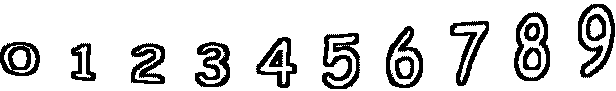16= 100   53= 8453= 76   24= 76   28= 121   24= 97 11= 42   38= 73   50= 126   72= 152